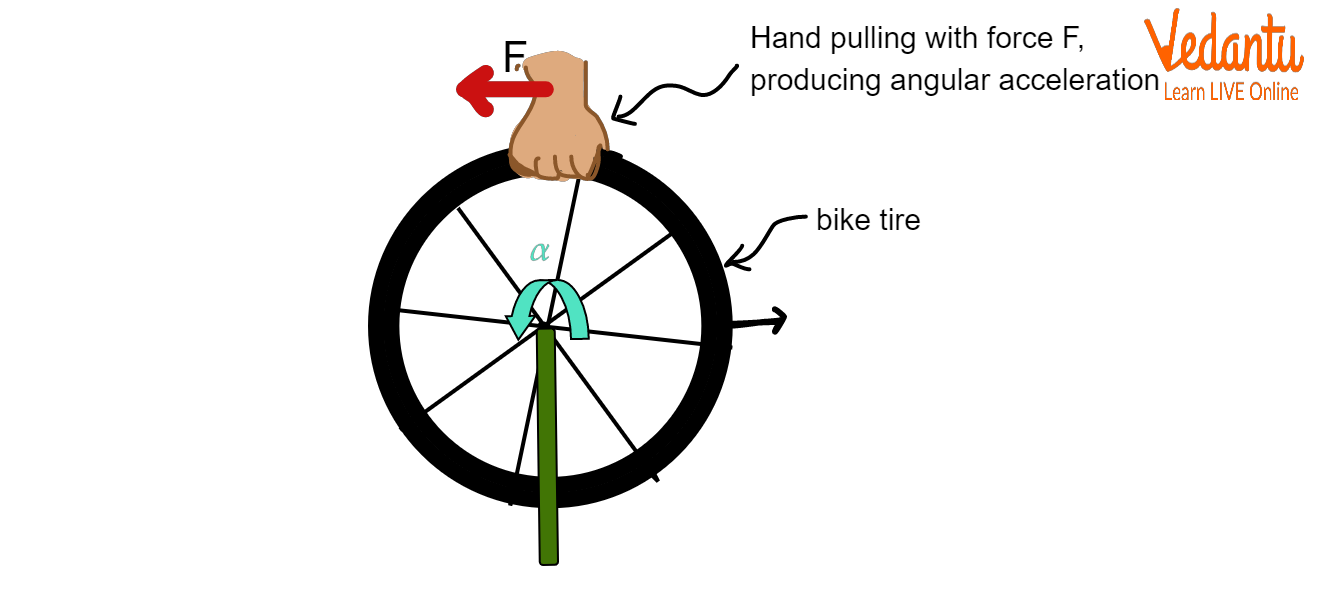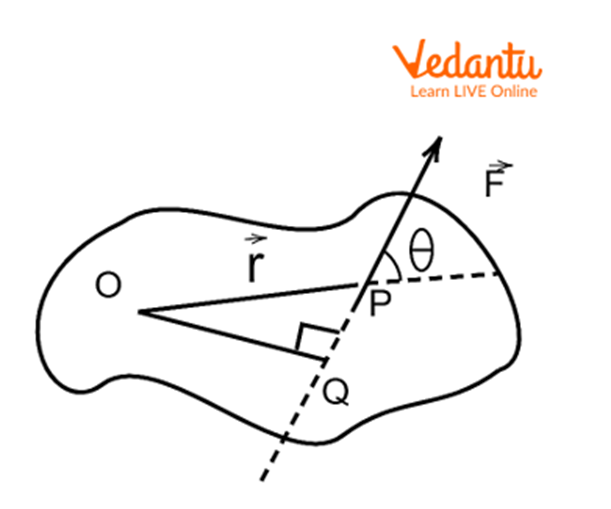Courses
Courses for Kids
Free study material
Offline Centres
More

# Moment of Force and Circular Motion for JEELast updated date: 01st Dec 2023
Total views: 21k
Views today: 0.21k## Definition of Rigid Body

A body can be called rigid if it does not deform under the action of external force. This means its geometrical shape and size will be unchanged. Thus, the distance between any arbitrary points lying on the body will always remain the same or there will be no change in the relative position of each particle. Under the application of any force (irrespective of its magnitude and direction), the shape will be unaltered. This is a hypothetical concept in actuality there exists no perfect rigid body, but when the deformation is negligible in a body then it can be considered a rigid body.

## Definition of Moment of Force and Circular Motion and Axis of Rotation

Here we will first describe the moment of force, circular motion and axis of rotation which explain the difference between force moment of force and circular motion. When a rigid body undergoes circular motion, all the particles lying on the axis of rotation remain stationary while all other particles move in a circle around the axis. When a rigid body that is free to move is acted upon by a force then it starts moving in the direction of the applied force.

If the direction of force is fixed the motion is called linear or translational motion. On the other hand, if the body is hinged or pivoted at a point, and the point of application of force is at some distance from the pivot then the body starts rotating about the fixed point( or the axis passing through the fixed point). This is known as circular motion. For example, when we turn the page of a book.Circular Motion

## Moment of Forces

In the case of translation motion, the linear acceleration is directly proportional to the applied force, whereas in the case of circular motion, angular acceleration is directly proportional to the product of force and the perpendicular distance between the line of application of force and the axis of rotation which is known as the moment of force or torque and the distance is known as the moment arm.

$\text{Magnitude of Moment}=\text{Force}\times \text{Moment Arm}$

It is denoted by $M$. Its SI unit is $N.m$. Its dimensions are $\left[ {{M}^{1}}{{L}^{2}}{{T}^{-2}} \right]$. It’s a vector quantity, whose direction is given by the Right-Hand Thumb Rule.

## Derivation for Moment of Force

Let us consider body with any shape that is capable of rotation about an axis which is passing through O and also it should be perpendicular to plane of paper: Assuming P is the point in the plane of paper, r is the position vector of point with respect to point O and F is the force on the body at point P that makes angle $\theta$ .Moment of Force on the Point in a Body

Considering right angle triangle PQO we have

$\sin \theta =\frac{OQ}{OP}$

$OQ=OP\sin \theta =r\sin \theta$

As we know the magnitude of moment of force $=Force\times Moment Arm$

$\therefore M=F\cdot OQ=F\cdot r\sin \theta$

$\therefore \overline{M}=\overrightarrow{r}\times \overrightarrow{F}$

So, we conclude that the vector product of the moment arm and the force is known as the moment of force whose direction will be determined by using the right-hand thumb rule. For the given case direction will be perpendicular to the plane of paper.

## Factors Affecting Moment of Force

As discussed above the moment of force is the product of two quantities, force and moment arm. Thus, the variation in these quantities varies at the moment of force. Let's take some examples also these are the moment of force examples

1. Lifting Heavy Masses Using a Lever

When we are required to lift a heavy mass, say a rock, we can use a lever. We apply forces on the long arm of the lever and the shorter arm placed under the rock. Since the moment of force due to the long arm will be larger thus heavy rocks can be lifted using smaller forces.

2. Why is it Easy to Open a Door by Pushing or Pulling at the Free End?

The door's axis of rotation passes through the hinges and the farthest point will be on the free end. By applying force on the free end we maximise the moment arm, thus larger moment of force acts on the door making it easy to open.

3. Use a Spanner to Loosen or Tighten a Nut.

A rusty nut is hard to lose and clearly, hands are not appropriate to do the task. But the use of a spanner reduces the effort drastically since the long arm of the spanner gives an appropriate position to apply force such that the moment of force acting is large enough to overcome friction resistance in nut and bolt assembly.

## Differences Between Force and Moment of Force

 Force Moment of Force When force is applied it results in changing the motion or path of the body Application of moment of force provides a circular or circular motion to a body It provides a linear acceleration to a body in general It provides an angular acceleration, which results in circular motion to the body which results in a circular motion around an axis Its SI unit is Newton denoted by $N$. Its SI unit is Newton-metre denoted by $N.m$. Force =  mass $\times$ acceleration Moment of force = Moment of mass Inertia $\times$ angular acceleration

## Conclusion

Torque and force measurement are both important to know when it comes to engineering. Torque always involves accelerations at an angle and it involves distance as well as force. The force is an action that causes some type of change in the motion of an object. There are many types of forces that need to be calculated including natural force, gravity, and electromagnetism.  When a body is free to move we are only concerned about forces but when the body is hinged about any point then torque and moment of inertia come into the picture and that needs to be calculated. In the case of force, the point of application is not relevant but in the case of a moment of force, the point of application is equally relevant as the magnitude of force.

## FAQs on Moment of Force and Circular Motion for JEE

1. Define the moment of force.

In the case of circular motion angular acceleration is directly proportional to the product of force and the perpendicular distance between the line of application of force and the axis of rotation which is known as the moment of Force or Torque and the distance is known as the moment arm. Also, moment of force is the force due which the turning effect of force on the body is generated about the axis.

2. Explain how we find the moment of force.

The product of the force applied and the distance from the axis is known as the moment of force.

Moment of Force = Moment of Mass Inertia x Angular Acceleration

And Newton metre is the SI unit of the moment of force. Moment of force is also expressed as

$M=F\times d$

Where F denotes the force applied in the system and d denotes the distance from the fixed point. This formula can be used to determine the moment of force balance as well as unbalanced forces.